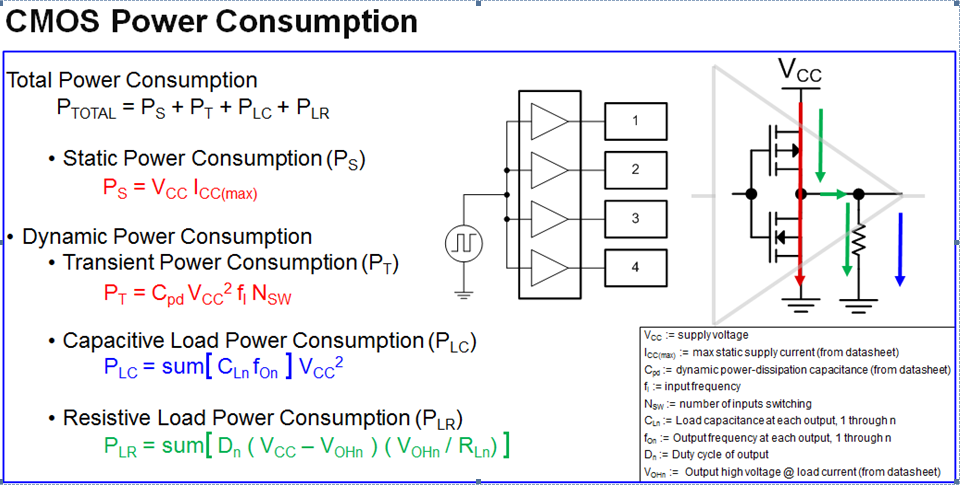If you have a related question, please click the "Ask a related question" button in the top right corner. The newly created question will be automatically linked to this question.

# [FAQ] How do I Calculate Power Consumption or Current Consumption for my CMOS Logic Device?

FAQ: Logic and Voltage Translation > Power and Thermals >> Current FAQ

CMOS logic devices are designed to be low powered. If you are interested in a low powered solution for your design, check out our AUP and HCS family of logic parts.

If you are interested in learning what the maximum power dissipation before thermal failure is, follow the FAQ link provided here:There are two components to determine the power consumption of your CMOS schematic:

• Static Power Consumption
• Dynamic Power Consumption

The following slide shows all the equations required to calculate both of these types of power consumption.Static power consumption:

CMOS Logic devices have very low static power consumption. This is the DC current consumption which the device consumes while it is on and no inputs are switching. Static power consumption is typically due to leakage currents and so it can be quickly calculated using the current flowing into the device, Icc. This value can be found in the datasheet for the Vcc you are operating at.

Dynamic Power Consumption:

• Transient Power: This is the product of Cpd (a number defined to help calculate transient power), Vcc of operation, the frequency your inputs are switching at and the number of inputs to your logic device.
• Capacitive and Resistive Load Consumption: This is not the power consumption of your load but the power loss due to the output FET stage of the logic device.

For a more detailed explanation, refer to the following appnote:

http://www.ti.com/lit/an/scaa035b/scaa035b.pdf

Here is an Excel calculator that you can quickly plug in the above values to calculate your power consumption:

Power Cosumption Calculator.xlsx